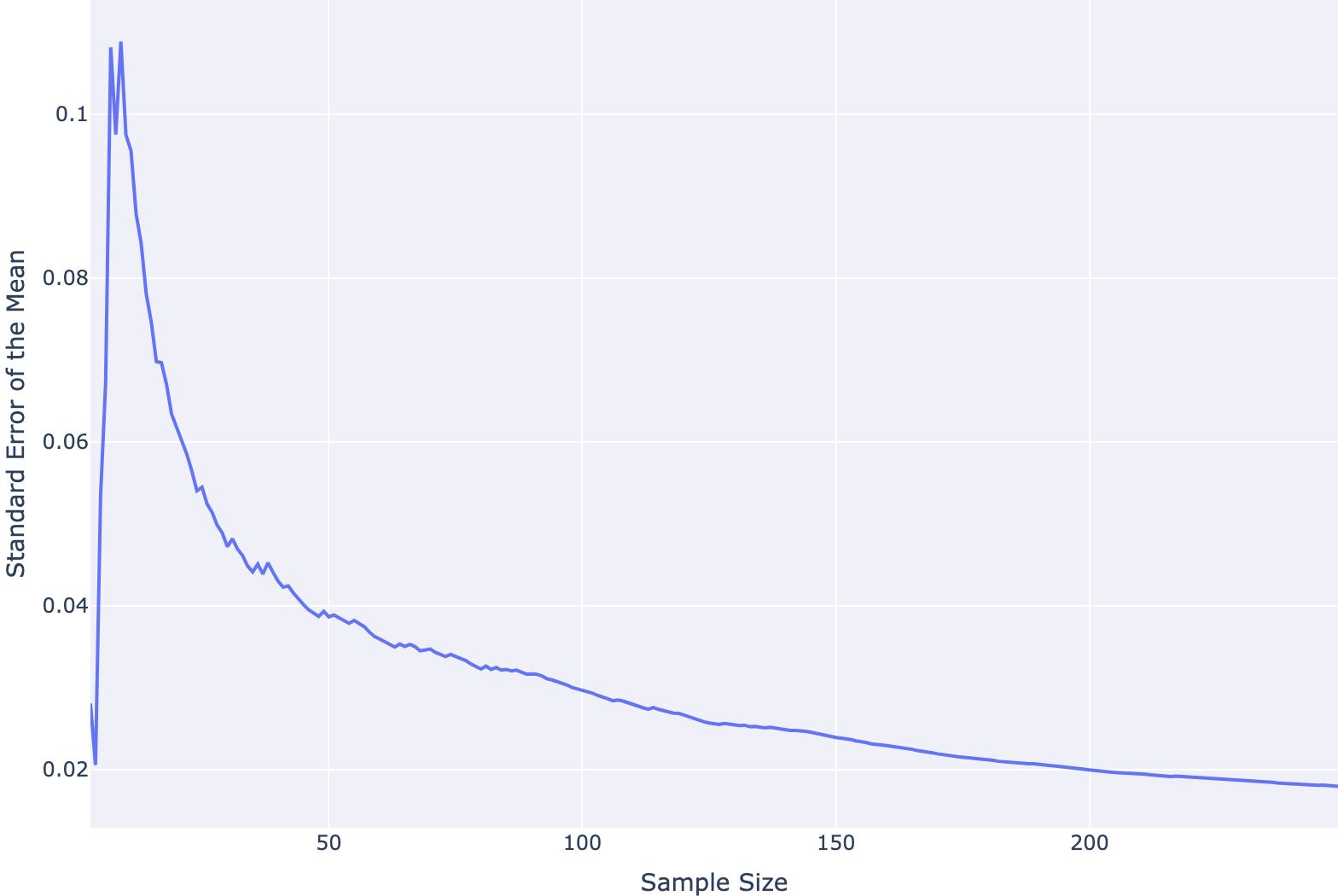## Data Analysis with Rust Notebooks

A practical book on Data Analysis with Rust Notebooks that teaches you the concepts and how they’re implemented in practice.

Get the book## Preamble

``````:dep darn = {version = "0.3.4"}
:dep ndarray = {version = "0.13.1"}
:dep ndarray-csv = {version = "0.4.1"}
:dep ureq = {version = "0.11.4"}
:dep ndarray-stats = {version = "0.3.0"}
extern crate csv;
extern crate ndarray;
extern crate noisy_float;

use std::io::prelude::*;
use std::fs::*;
use ndarray::prelude::*;
use std::str::FromStr;
use noisy_float::types::n64;
use ndarray_stats::{QuantileExt, interpolate::Nearest, interpolate::Midpoint};
``````

## Introduction

In this section, we're going to take a look at some of the tools we have for descriptive statistics. Some of these are built into the `ndarray` crate that we're already familiar with, but some of them require another crate, `ndarray-stats`. This crate provides more advanced statistical methods for the array data structures provided by `ndarray`.

The currently available methods include:

• Order statistics (minimum, maximum, median, quantiles, etc.);
• Summary statistics (mean, skewness, kurtosis, central moments, etc.)
• Partitioning;
• Correlation analysis (covariance, pearson correlation);
• Measures from information theory (entropy, KL divergence, etc.);
• Measures of deviation (count equal, L1, L2 distances, mean squared err etc.);
• Histogram computation.

For now, we'll focus on the first few methods we would normally use when interrogating a numerical dataset, e.g. central tendency and variance.

We will continue using the Iris Flower dataset, so we need to load it into our raw string array first.

``````let file_name = "Iris.csv";

let res = ureq::get("https://datacrayon.com/datasets/Iris.csv").call().into_string()?;

let mut file = File::create(file_name)?;
file.write_all(res.as_bytes());
remove_file(file_name)?;

let data: Array2<String>= rdr.deserialize_array2_dynamic().unwrap();
let mut headers : Vec<String> = Vec::new();

};
``````

### Moving Data to Typed Arrays

We need to convert from String to the desired type, and move our data over to the typed arrays.

``````let mut features: Array2::<f32> =  Array2::<f32>::zeros((data.shape(),0));

for &f in [1, 2, 3, 4].iter() {
features = ndarray::stack![Axis(1), features,
data.column(f as usize)
.mapv(|elem| f32::from_str(&elem).unwrap())
.insert_axis(Axis(1))];
};

let labels: Array1::<String> = data.column(5).to_owned();
``````

## Descriptive Statistics

Descriptive statistics help us summarize a given representation of a dataset. We can divide these into two areas: measures of central tendency (e.g. mean and median) and measures of variance (e.g. standard deviation and min/max values). Let's have a look at how we can calculate these using a combination of `ndarray` and `ndarray-stats`.

### Measures of Central Tendency

#### Mean

Calculating the mean is one of the basic methods provided by `ndarray`. We can calculate the arithmetic mean of all elements in our array using `.mean()`

``````features.mean().unwrap()
``````
`3.463667`

In the case of our two-dimensional array, we are more likely interested in the mean across one of our axes. To find the mean of each column we can use `.mean_axis()`.

``````println!("{}", features.mean_axis(Axis(0)).unwrap());
``````
```[5.8433347, 3.054, 3.7586665, 1.1986669]
```

#### Median

Calculating the median is not provided by `ndarray`, so this is where we start turning to `ndarray-stats`. We can use the `.quantile_axis_mut()` function provided by `ndarray-stats` with a parameter setting of `q=0.5` to return our median across a 1-dimensional lane, let's try this for the first column of our dataset.

``````features.column(0).to_owned()
.quantile_axis_skipnan_mut(
Axis(0),
n64(0.5),
&ndarray_stats::interpolate::Linear)
.unwrap().into_scalar()
``````
`5.8`

This works well, but it would be nice to have an output similar to `.mean_axis()` where we calculate the median for each column and output as a vector. For this, we can make use of iterators provided by `ndarray`, `.axis_iter()`.

``````features.axis_iter(Axis(1)).map(|elem| elem.to_owned()
.quantile_axis_skipnan_mut(
Axis(0),
n64(0.5),
&ndarray_stats::interpolate::Linear)
.unwrap().into_scalar()).collect::<Vec<_>>()
``````
`[5.8, 3.0, 4.3500004, 1.3]`

### Measures of Variability

#### Variance

To calculate the variance (computed by the Welford one-pass algorithm), we can use `.var_axis()` provided by `.ndarray()`. The delta degrees of freedom parameter, `ddof`, determines whether we calculate the population variance (`ddof = 0`), or the sample variance (`ddof = 1`).

With this, we can calculate the population variance for each column.

``````println!("{}", features.var_axis(Axis(0), 0.0));
``````
```[0.6811211, 0.18675052, 3.0924246, 0.57853156]
```

Similarly, we can calculate the sample variance for each column.

``````println!("{}", features.var_axis(Axis(0), 1.0));
``````
```[0.68569237, 0.18800387, 3.1131792, 0.5824143]
```

#### Standard Deviation

The standard deviation, `.std_axis()`, is calculated from the variance (again with the Welford one-pass algorithm) and works in a similar way to `.var_axis()`. The delta degrees of freedom parameter, ddof, determines whether we calculate the population standard deviation (`ddof = 0`), or the sample standard deviation (`ddof = 1`).

With this, we can calculate the population standard deviation for each column.

``````println!("{}", features.std_axis(Axis(0), 0.0));
``````
```[0.82530063, 0.4321464, 1.7585291, 0.7606126]
```

Similarly, we can calculate the sample standard deviation for each column.

``````println!("{}", features.std_axis(Axis(0), 1.0));
``````
```[0.82806545, 0.43359414, 1.7644204, 0.76316077]
```

#### Minimum and Maximum Values

For the minimum and maximum values across each column we will turn to `ndarray-stats`. We can use the `.quantile_axis_mut()` function again different parameter settings for `q` to return our minimimum and maximum values across 1-dimensional lanes. Let's pair this approach with `.axis_iter()` once again to calculate the values across multiple columns.

#### Minimum Value

To calculate the minimum value for each column we will need to use `q = 0.0`.

``````features.axis_iter(Axis(1)).map(|elem| elem.to_owned()
.quantile_axis_skipnan_mut(
Axis(0),
n64(0.0),
&ndarray_stats::interpolate::Linear)
.unwrap().into_scalar()).collect::<Vec<_>>()
``````
`[4.3, 2.0, 1.0, 0.1]`

#### Maximum Value

To calculate the maximum value for each column we will need to use `q = 1.0`.

``````features.axis_iter(Axis(1)).map(|elem| elem.to_owned()
.quantile_axis_skipnan_mut(
Axis(0),
n64(1.0),
&ndarray_stats::interpolate::Linear)
.unwrap().into_scalar()).collect::<Vec<_>>()
``````
`[7.9, 4.4, 6.9, 2.5]`

## Conclusion

In this section, we had a look at some of the tools we have available for descriptive statistics. We used some of the basic functionality provided by `ndarray`, and turned to `ndarray-stats` for the more advanced functionality when we needed to.

## Data Analysis with Rust Notebooks

A practical book on Data Analysis with Rust Notebooks that teaches you the concepts and how they’re implemented in practice.

Get the book

## ISBN

978-1-915907-10-3

## Cite

Rostami, S. (2020). Data Analysis with Rust Notebooks. Polyra Publishing.